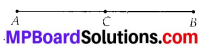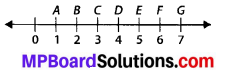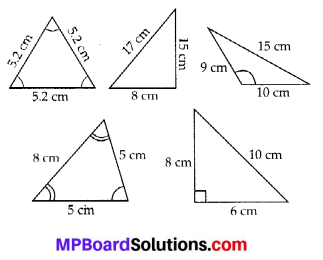## MP Board Class 6th Maths Solutions Chapter 5 Understanding Elementary Shapes Ex 5.1

Question 1.
What is the disadvantage in comparing line segments by mere observation?
Solution:
There may be chance of error due to improper viewing.

Question 2.
Why is it better to use a divider than a ruler, while measuring the length of a line segment?
Solution:
It is better to use a divider than a ruler, because the thickness of the ruler may cause difficulties in reading of the length of a line segment. However, a divider gives accurate measurement.Question 3.
Draw any line segment, say $$\overline{A B}$$. Take any point C lying in between A and B. Measure the lengths of AB, BC and AC. Is AB = AC + CB? [Note : If A, B, C are any three points on a line such that AC + CB = AB, then we can be sure that C lies between A and B.]
Solution:AB = 4 cm,
AC = 2 cm,
CB = 2 cm
Hence, AC + CB = 2 cm + 2 cm = 4 cm = AB
Yes, AB = AC + CB.Question 4.
If A, B, C are three points on a line such that AB = 5 cm, BC = 3 cm and AC =8 cm, which one of them lies between the other two?
Solution:
Since, $$\overline { AC }$$ is the longest line segment. Thus B is the point lying between A and C.

Question 5.
Verify, whether D is the mid point of AG.Solution:
DG = 3 units,
Thus, D is the mid-point of AG.Question 6.
If B is the mid point of $$\overline { AC }$$ and C is the mid point of $$\overline { BD }$$, where A, B, C, D lie on a straight line, say why AB = CD?
Solution:
B is the mid point of $$\overline { AC }$$.
∴ AB = BC … (i)
And C is the mid point of BD.
∴ BC = CD … (ii)
From (i) and (ii), we get
AB = CDQuestion 7.
Draw five triangles and measure their sides. Check in each case, if the sum of the lengths of any two sides is always less than the third side.
Solution:
Sum of the lengths of any two sides of a triangle can never be less than length of the third side.